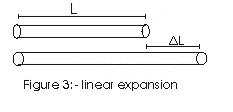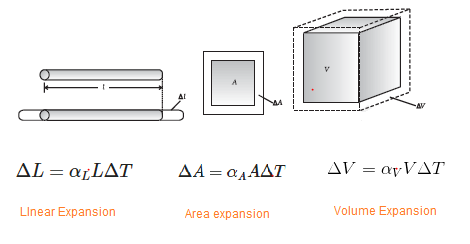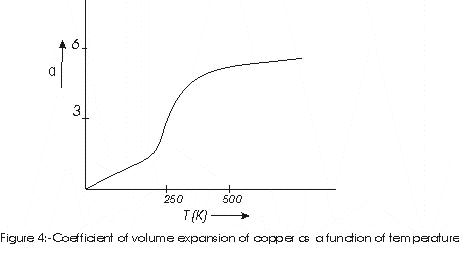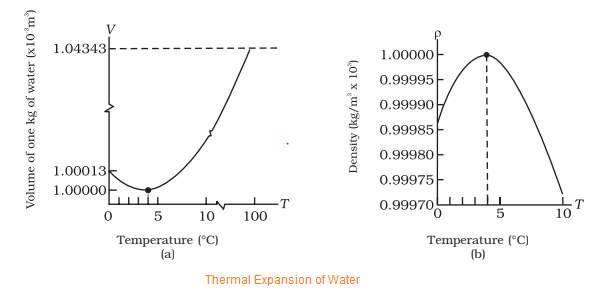# Thermal expansion

## Thermal Expansion

Most of the solid material expands when heated. Increase in dimension of a body due to increase in its temperature is called thermal expansion. For small change in temperature $\Delta T$ of a rod of length $L$, the fractional change in length $\frac{\Delta L}{L}$ is directly proportional to $\Delta T$(Fig 3)\begin{equation} \frac{\Delta L}{L}=\alpha_{L}\Delta T \tag{2} \end{equation} or, \begin{equation} \Delta L=\alpha_{L}L\Delta T \tag{3} \end{equation} Constant $\alpha_L$ characterizes the thermal expansion properties of a particular material and it is known as coefficient of linear expansion. For materials having no preferential direction, every linear dimension changes according to equation (3) and $L$ could equally well represent the thickness of the rod, side length of the square sheet etc. Normally metals expand more and have high value of $\alpha$. Again consider the initial surface area $A$ of any surface and $A'$ is the area of the solid when the temperature of the body changes by $\Delta T$ then increase in surface area is given by \begin{equation} \Delta A =\alpha_{A}A\Delta T \tag{4} \end{equation} where $\alpha_{A}$ is the coefficient of area expansion.
Similarly, we can define coefficient of volume expansion as fractional change in volume $\frac{\Delta V}{V}$ of a substance for a temperature change $\Delta T$ as \begin{equation} \alpha_{V}=\frac{\Delta V}{V\Delta T} \tag{5} \end{equation}$K^{-1}$ is the unit of these coefficients of expansions. These three coefficient are not strictly constant for a substance and there value is depends on temperature range in which they are being measured. As an example, figure below shows that coefficient of volume expansion increase with temperature and takes a constant value above $500K$### Relation between volume and linear coefficient of expansion for solid material:

Consider a solid parallelepiped with dimension $L_1$,$L_2$ and $L_3$ then its volume is $$V= L_{1}L_{2}L_{3}$$ When temperature increase by a amount $\Delta T$ then each linear dimension changes and then new volume is \begin{align*} V+\Delta V &=L_{1}L_{2}L_{3}(1+\alpha_{L}\Delta T)^{3}\\ &= V(1+\alpha_{L}\Delta T)^{3}\\ &= V(1+3\alpha_{L}\Delta T+3\alpha_{L}2(\Delta T)^{2}+\alpha_{L}3(\Delta T)^{3}) \end{align*} if $\Delta T$ is small then higher order of $\Delta T$ can be neglected. Thus we find $$V+\Delta V=V(1+3\alpha_{L}\Delta T)$$ or, $$\Delta V=3\alpha_{L}V_{0}\Delta T$$ Comparing this with equation (5) we find $$\alpha_{V}=3\alpha_{L}$$

### Thermal Expansion of Water

• Water exhibits an anomalous behaviour.
• The volume of the water decreases as temperature increases from 0 °C and 4 °C. The volume of a given amount of water increases as the temperature increases further of 4 °C
• So, water has a maximum density at 4 °C.
• This anomalous behaviour has the favourable effect for animals living in water. Since the density of the water is maximum at 4°C, water at the bottom of the lakes remains at 4 °C in winter even if the surface freezes. This allow marine life to survive. If water did not have this property, lakes and ponds would freeze from the bottom up, which would destroy much of their animal and plant life.## Solved Examples

Question 1
A blacksmith fixes iron ring on the rim of the wooden wheel of a bullock cart. The diameter of the rim and the iron ring are 5.243 m and 5.231 m respectively at 27 °C. To what temperature should the ring be heated so as to fit the rim of the wheel? ( $\alpha =1.20 \times 10^{-5} C^{-1}$)
Solution
Given,
$T_1 = 27 ^0$ C
$L_1 = 5.231$ m
$L_2 = 5.243$ m
So,
$L_2 =L_1 [1+ \alpha (T_2 - T_1)]$
$5.243 m = 5.231 m [1 + 1.20 \times 10^{-5} (T_2 - 27)]$
or $T_2 = 218 ^0$C.

Question 2
An iron ring has to fit over a cylindrical rod. The diameter of the rod and iron ring are 6.445 cm and 6.420 cm at 20 ° C.The ring can slip over the iron rod only if the diameter of the ring is about .008 cm larger than iron rod.To what temperature should the ring be heated so as to fit the cylindrical rod? ( $\alpha =1.20 \times 10^{-5} C^{-1}$)
Solution
Given,
$T_1 = 20 ^0$ C
$L_1 = 6.420$ m
$L_2 = 6.445 + .008=6.453$ m
So,
$L_2 =L_1 [1+ \alpha (T_2 - T_1)]$
$6.453 m = 6.420 m [1 + 1.20 \times 10^{-5} (T_2 - 20)]$
or $T_2 = 450 ^0$C.

Question 3
when a circular disc having hole is heated. if the hole size increase or decreases?
Solution
The hole size increases

Question 4
When the lid of the glass jar is tight, we often put it a hot water and then it is easy to open. Why?
Solution
The metal expands on heating. You might say , glass also expands ,but metal expands more than glass for same temperature difference. So it becomes easy to open